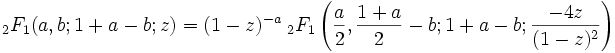# Hypergeometric transformations and identities

DavidSmith
How do you derive hypergeometirc identities of the form

2F1(a,b,c,z)= gamma function. What I mean is that the hypergeometric function converges to a set of gamme functions function in terms of (a,b,c)

where z is not 1,-1, or 1/2 ?

The hypergeometric identities in the mathworld summary which give gamma functions only have values of 1,-1, and 1/2 for Z but none others.

I have seen hypergeometric functions where z=1/8,1/3, 2/3 etc that give gamma functions but have no idea how to deive them despite many months of time and research.

DavidSmith
After spending another 3 days with the problem I used a kummer quadratic identity combined with annother kummer transformation to get a hypergeometric function for z=-1/8(8^(-3b+1)/2)*2F1((3b-1)/2,-b/2+1/2;1/2+b;-1/8)=

4*(gammafunction(1/2)*gammafunction(2b))/(b*gammafunction(b/2))^2

there are more possible

Last edited: